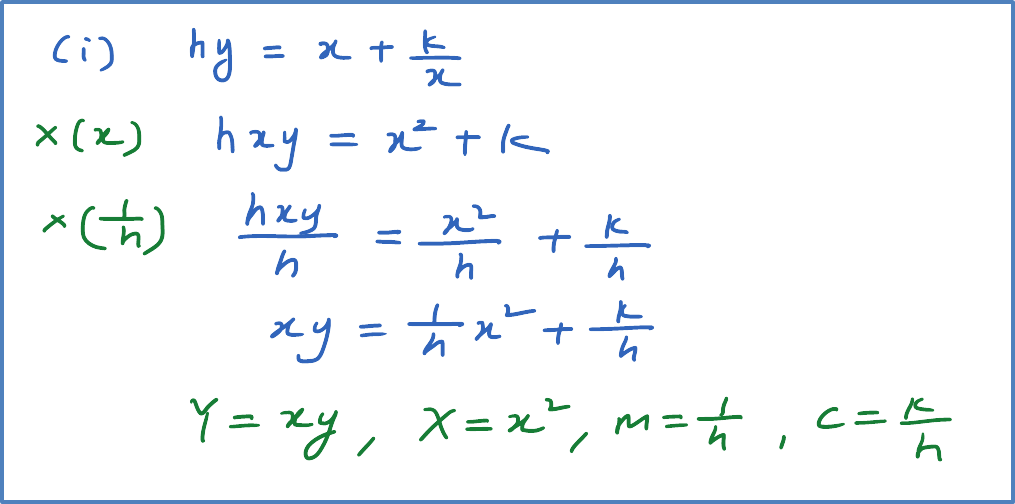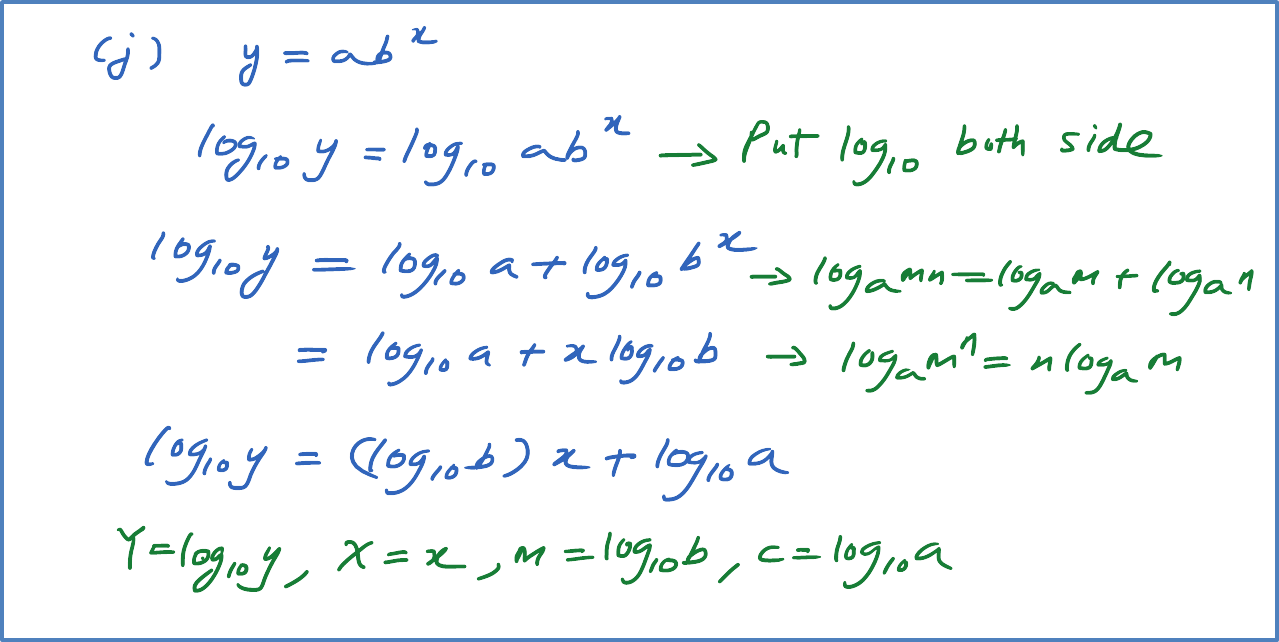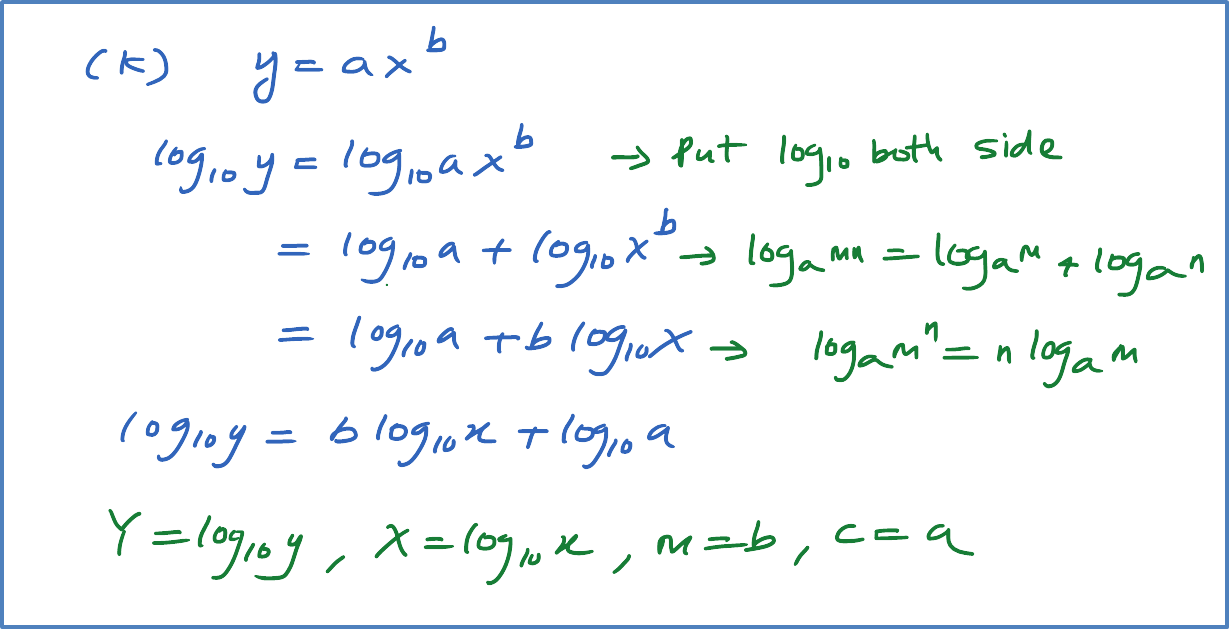# 2.5.2 Reduce Non-Linear Function to Linear Function – Examples (g) to (l)

Examples:
Reduce each of the following equations to the linear form. Hence, state the gradient and the Y-intercept of the linear equations in terms of  a and b.
(g)  $k{x}^{2}+t{y}^{2}=x$
(h)  $y=\frac{x}{p+qx}$
(i)  $hy=x+\frac{k}{x}$
(j)  $y=a{b}^{x}$
(k)  $y=a{x}^{b}$
(l)  $y=a{b}^{x+1}$

[Note :
X and Y cannot have constant, but can have the variables (for example x and y)
m and c can only have the constant (for example a and b), cannot have the variables x and y]

Solution: